# How to Divide a Decimal by a Decimal

How to Divide a Decimal by a Decimal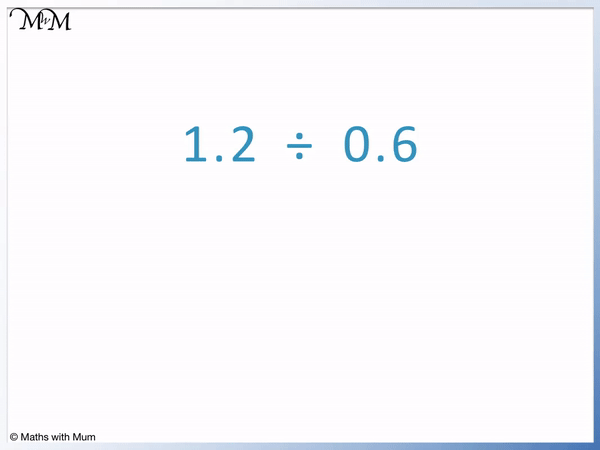• To divide a decimal by another decimal, first make the number after the division sign a whole number.
• We do this by multiplying it by 10, 100, 1000 etc., moving the decimal point to the right.
• We multiply the number before the division sign by the same amount, moving its decimal point the same number of places.
• For example in 1.2 ÷ 0.6, 0.6 can be multiplied by 10 to change it into 6.
• We therefore also multiply 1.2 by 10 to make it 12.
• 1.2 ÷ 0.6 is changed into 12 ÷ 6.
• 12 ÷ 6 = 2 and so, 1.2 ÷ 0.6 = 2. The answer is 2.
• The number of digits after the decimal point in the number after the division sign is the same as the number of zeros in the number we multiplied by.
• For example, 0.6 has 1 digit after the decimal point and so we multiplied it by 10, which has 1 zero in it.
Multiply both numbers by 10, 100, 1000 etc. so that the number being divided by is not a decimal. Then do the division.• Here is 5.72 ÷ 0.4.
• 0.4 has 1 digit after the decimal point and so, we multiply it by 10 which has 1 zero in it.
• We must also multiply 5.72 by 10 to keep the answer the same.
• 5.72 ÷ 0.4 becomes 57.2 ÷ 4.
• We can work out this division using short division, dividing each digit of 57.2 by 4.
• 5 ÷ 4 = 1, remainder 1. We write the remainder next to the 7 to make 17
• 17 ÷ 4 = 4, remainder 1. We write the remainder next to the 2 to make 12.
• 12 ÷ 4 = 3 exactly. There is no remainder so we stop.
• 57.2 ÷ 4 = 14.3 and so, 5.72 ÷ 0.4 = 14.3.
• The decimal point in the answer is written directly above the decimal point of the number in the division.Supporting Lessons

#### Dividing Decimals with Remainders# Dividing Decimals by Decimals

## How to Divide Decimals by Decimals Step by Step

To divide decimals by decimals, follow these steps:

1. Look at the number after the division sign and move its decimal point to the right to make it a whole number.
2. Move the decimal point in the number before the division sign by the same number of places.
3. Divide these new numbers using short division.

For example, in 0.35 ÷ 0.007, the decimal point is moved 3 places right in both numbers to make the division become 350 ÷ 7.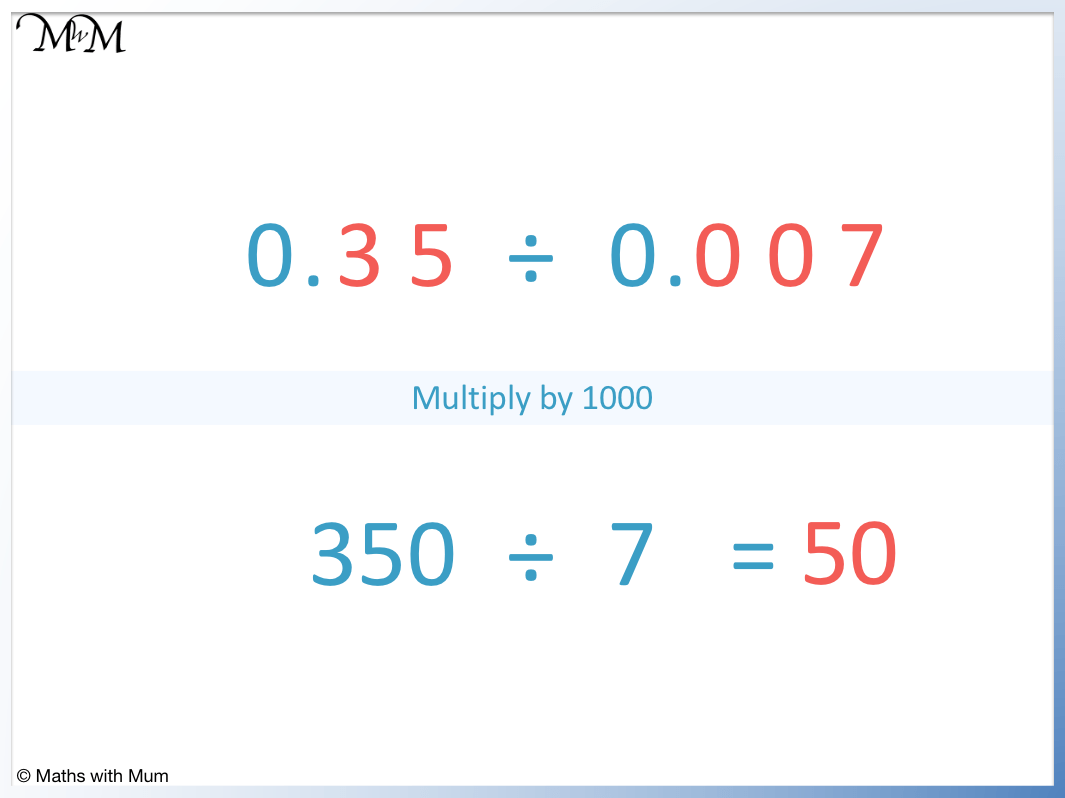350 ÷ 7 = 50 and so, 0.35 ÷ 0.007 also is equal to 50.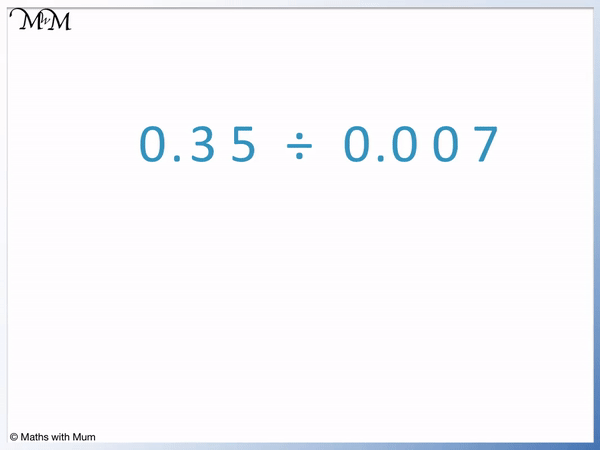## Dividing Decimals without Remainders

2.4 ÷ 0.03 is an example of dividing decimals where there are no remainders. 0.03 is multiplied by 100 to make it become 3 and 2.4 is multiplied by 100 to make it become 240. 240 ÷ 3 = 80 and so, 2.4 ÷ 0.03 = 80. This answer is exact and contains no remainders.0.03 is multiplied by 100 to make it a whole number. This is because 100 has 2 zeros in it and there are 2 digits after the decimal point in 0.03.

We must also multiply 2.4 by 100 to keep the answer the same. 2.4 multiplied by 100 is 240.

We can now do the division. 240 ÷ 3 = 80 and so, 2.4 ÷ 0.03 is also 80.

## Dividing Decimals with Remainders

0.561 ÷ 0.06 is an example of dividing decimals involving remainders. Both 0.06 and 0.561 are multiplied by 100 to make the division become 56.1 ÷ 6. We then divide digit by digit. 6 does not divide into 5 and so, the 5 is carried as a remainder to make 56. 56 ÷ 6 = 9, remainder 2. The 2 is carried over to the 1 to make 21. 21 ÷ 6 = 3, remainder 3. Another zero must be added and the remainder of 3 is carried over to make 30. 30 ÷ 6 = 5 and the division is complete. 0.561 ÷ 0.06 = 9.35.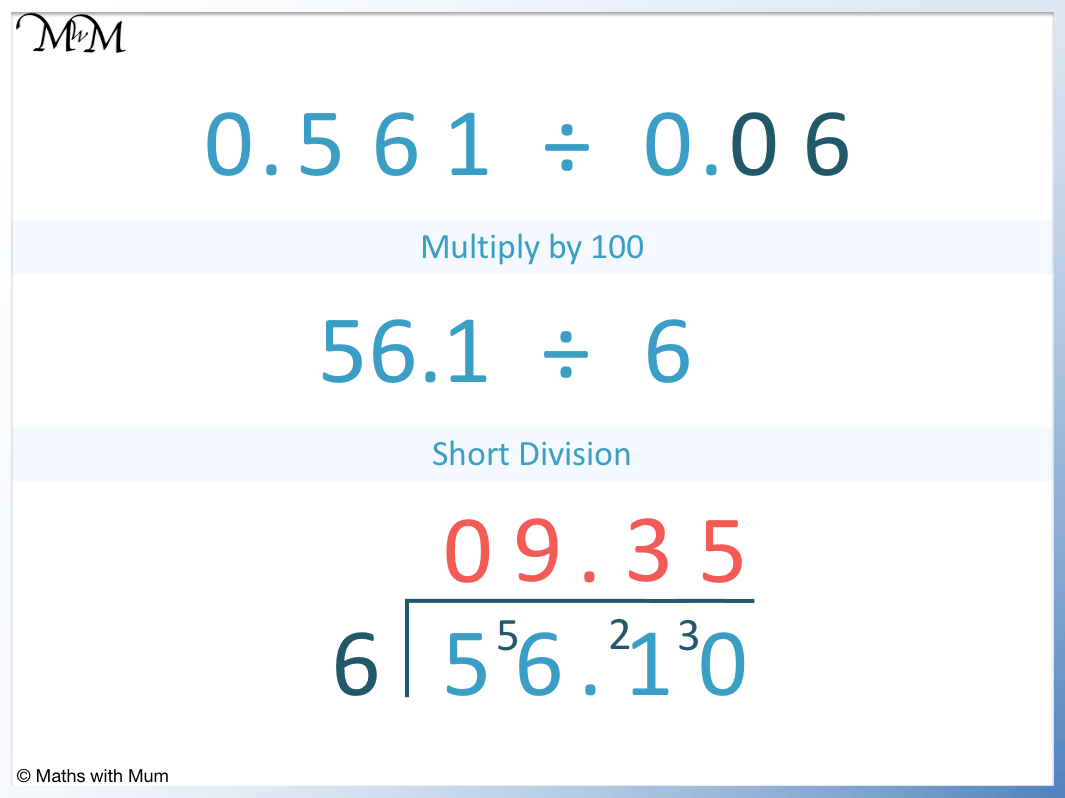0.06 is multiplied by 100 to make it a whole number of 6. This is because 0.06 contains 2 digits after the decimal point and 100 contains 2 zeros.

We also multiply 0.561 by 100 by moving the decimal point 2 places to the right, making 56.1.

56.1 ÷ 6 can be done using short division. We divide each digit in 56.1 by 6 individually.## Dividing Decimals Examples

2.0223 ÷ 0.003 is an example of dividing decimals. To make 0.003 a whole number, we multiply it by 1000 since 0.003 contains 3 digits after the decimal point and 1000 contains 3 zeros. We also multiply 2.0223 by 1000 by moving the decimal place 3 places right to become 2022.3. We then divide 2022.3 by 3 using short division to get an answer of 674.1.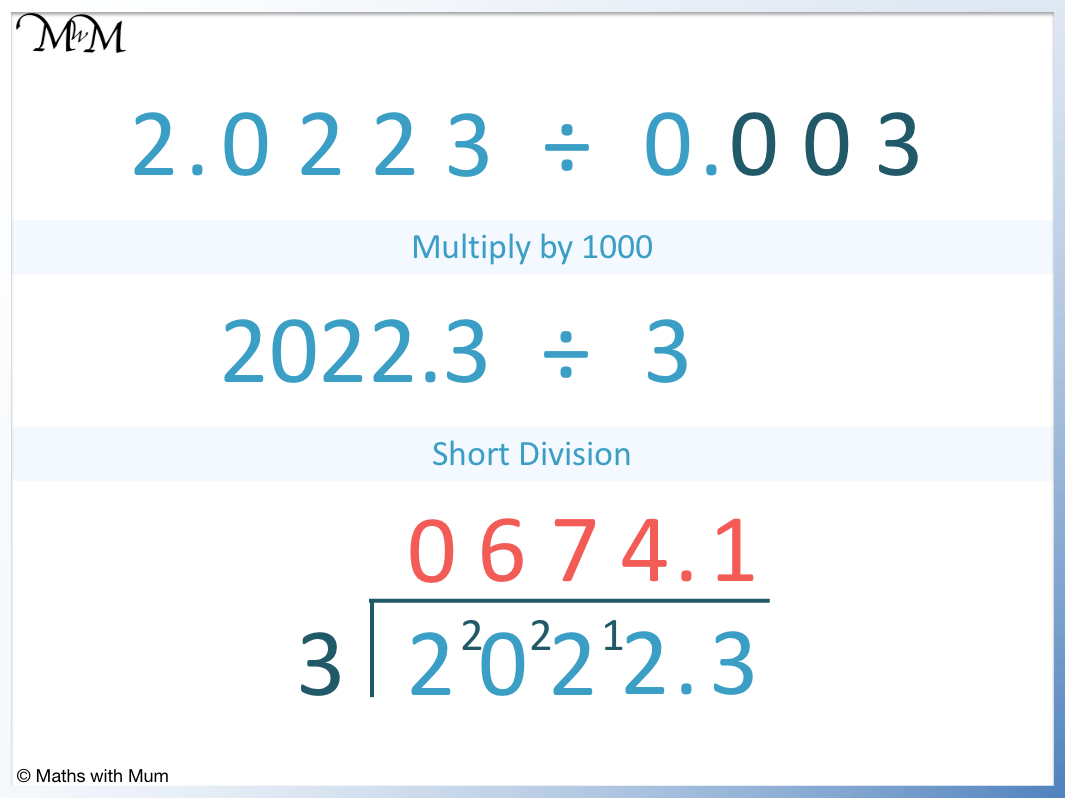2022.3 is divided using short division by dividing each digit individually by 3 from left to right.

2 ÷ 3 = 0, remainder 2. We carry the 2 over to the 0 to make 20.

20 ÷ 3 = 6, remainder 2. We carry the 2 over to the 2 to make 22.

22 ÷ 3 = 7, remainder 1. We carry the 1 over to the 2 to make 12.

12 ÷ 3 = 4. We write the decimal point after this digit, directly above the decimal point in the example.

Finally, 3 ÷ 3 = 1.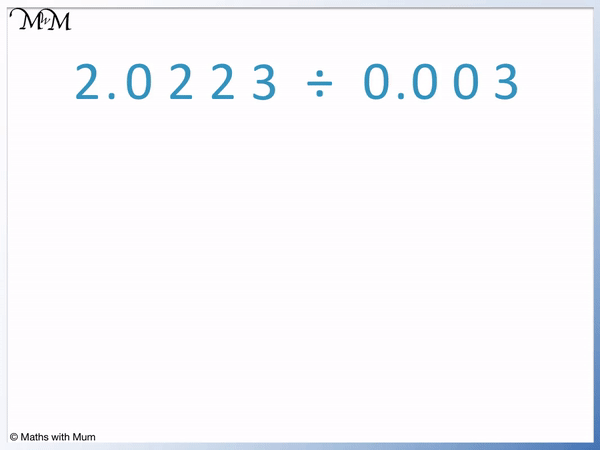20223 ÷ 3 = 674.1 and so, 2.0223 ÷ 0.003 = 674.1.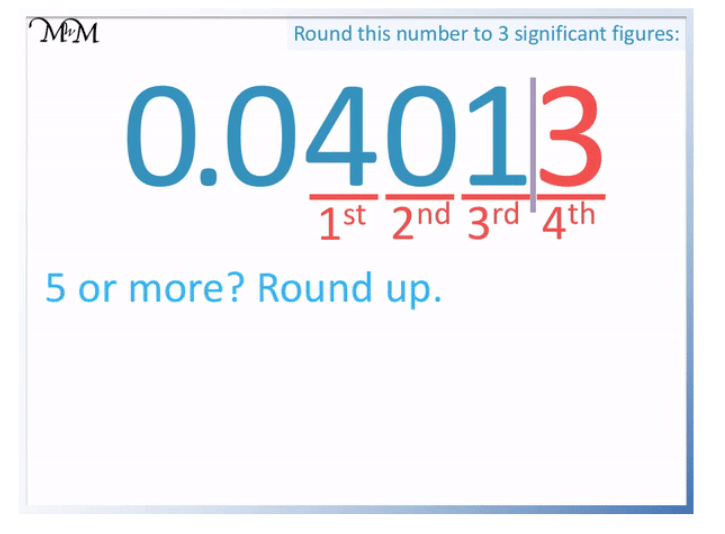Now try our lesson on Multiplying Decimals where we learn how to multiply 2 decimal numbers.